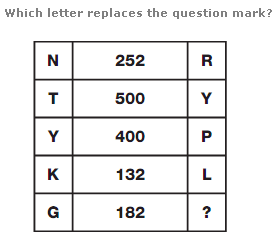# Logical Puzzle With AnswerWhich letter replaces the question mark?

N 252 R

T 500 Y

Y 400 P

K 132 L

G 182 ?

Try solving this puzzle. If you cannot solve it then scroll down to find the answer.

SCROLL DOWN TO FIND THE ANSWER

 Answer : Z Explanation : In each row, multiply the numerical values of the left and right hand letters, putting the result in the centre.

N(14) 252 R(18)

T(20) 500 Y(25)

Y(25) 400 P(16)

K(11) 132 L(12)

G(7) 182(7*26) Z(26)

Keywords:

Logical puzzles,
Logical puzzle with answer,
Logical and reasoning puzzles with answer,
Logical and reasoning puzzles,
Riddles and puzzles,
logical riddles and puzzles with answer